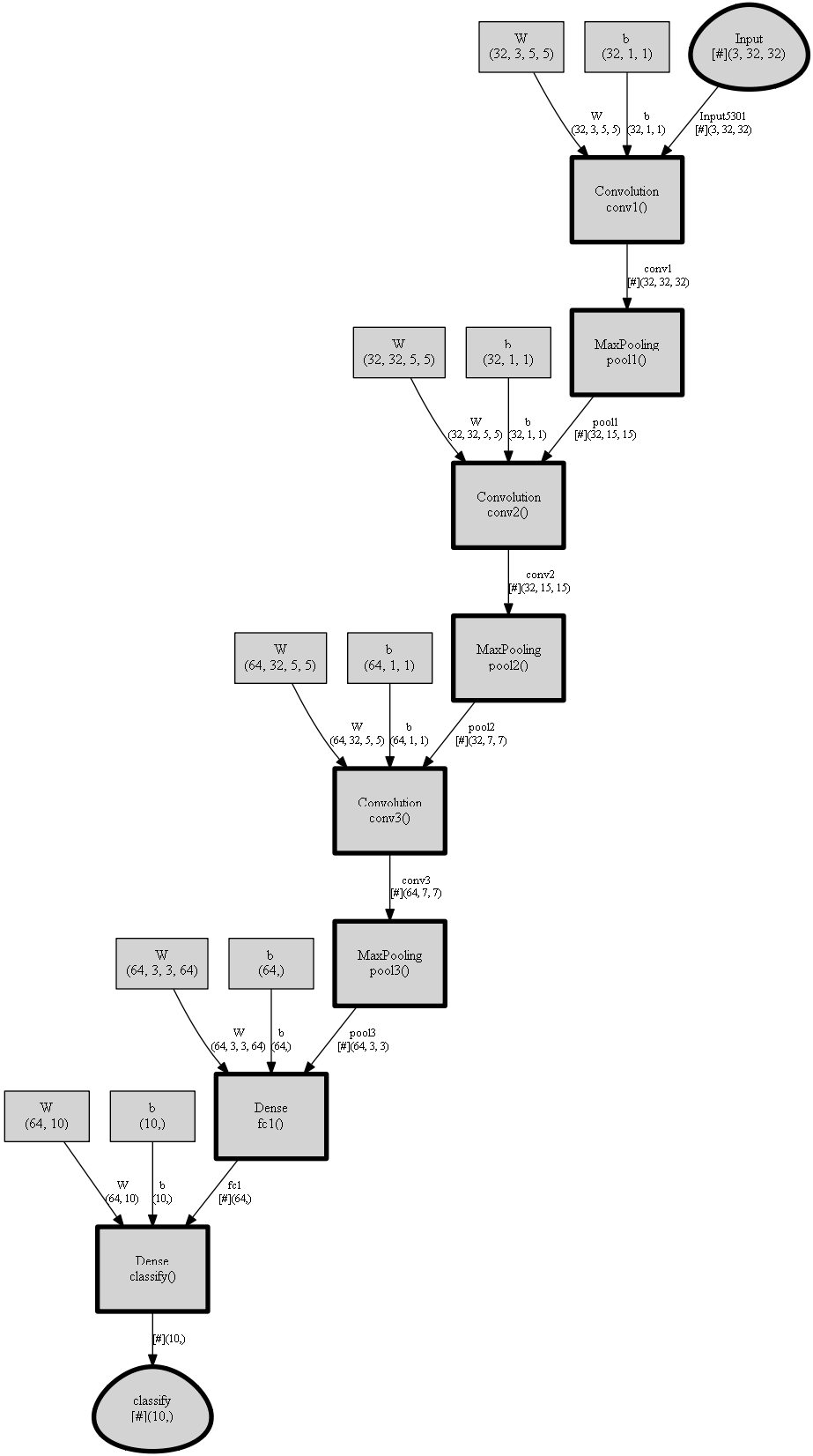# Using Graphviz for Visualization

CNTK provides a simple way to visualize the underlying computational graph of a model using Graphviz, an open-source graph visualization software.

To illustrate a use case, let's first build a simple convolutional network using the CNTK Layers library.

``````import cntk as C

def create_model(x, num_classes):
with C.layers.default_options(init=C.glorot_uniform(), activation=C.relu):
model = C.layers.Sequential([
C.layers.For(range(3), lambda i: [
C.layers.Convolution((5,5), [32,32,64][i], pad=True, name=['conv1', 'conv2', 'conv3'][i]),
C.layers.MaxPooling((3,3), strides=(2,2), name=['pool1', 'pool2', 'pool3'][i])
]),
C.layers.Dense(64, name='fc1'),
C.layers.Dense(num_classes, activation=None, name='classify')
])
return model(x)
``````

Now assuming we are training on the CIFAR-10 dataset, which consists of 32x32 images in 10 classes, we can assign the input shape correspondingly. Refer to the CNTK 201A tutorial for instructions on downloading and preparing the CIFAR-10 dataset for use in CNTK.

``````input_var = C.input_variable((3,32,32))
z = create_model(input_var)
``````

To get the underlying description of the computational graph, CNTK provides a `plot` function in the `cntk.logging.graph` module. The `plot(root, filename=None)` function returns a network description of the graph starting at the `root` node provided. In addition, if `filename` is specified, the method outputs a DOT, PNG, PDF or SVG file (corresponding to the filename suffix).

In order to output the DOT output, you will need to install `pydot-ng` (`pip install pydot_ng`). And if you would like PNG, PDF or SVG output, you will need Graphviz in addition to `pydot-ng`. Once you've installed Graphviz, ensure that the Graphviz binaries are in your PATH environment variable.

``````import pydot_ng

graph_description = C.logging.graph.plot(z, "graph.png")
print(graph_description)
``````
``````Convolution(Parameter5302, Parameter5303, Input5301) -> Block6011_Output_0;

MaxPooling(Block6011_Output_0) -> Block6023_Output_0;

Convolution(Parameter5332, Parameter5333, Block6023_Output_0) -> Block6039_Output_0;

MaxPooling(Block6039_Output_0) -> Block6051_Output_0;

Convolution(Parameter5362, Parameter5363, Block6051_Output_0) -> Block6067_Output_0;

MaxPooling(Block6067_Output_0) -> Block6079_Output_0;

Dense(Parameter5710, Parameter5711, Block6079_Output_0) -> Block6095_Output_0;

Dense(Parameter5730, Parameter5731, Block6095_Output_0) -> Block6110_Output_0;
``````

If you are using a Jupyter Notebook, you can display the graph visualization output inline:

``````from IPython.display import Image

display(Image(filename="graph.png"))
``````For a more detailed example of visualization using `logging.graph.plot`, refer to the How to debug manual.